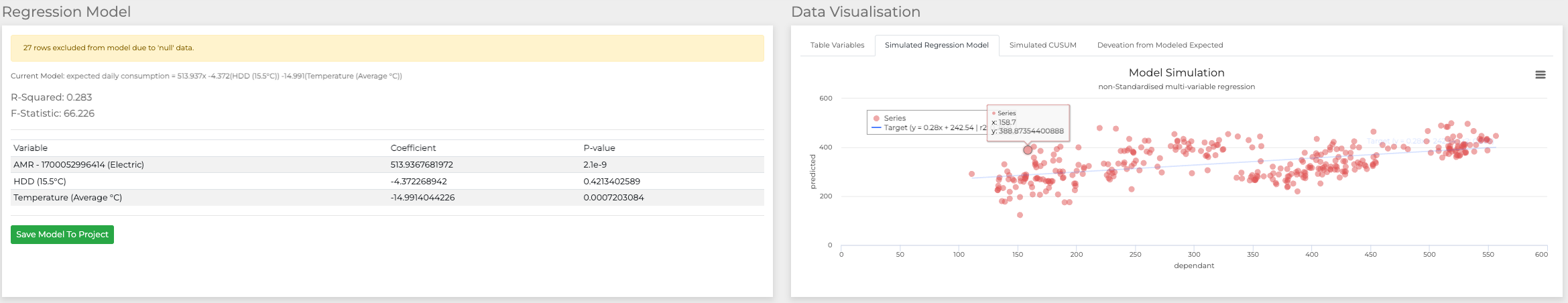# Regression

### Regression

Multi-variable linear regression is a statistical method used to understand and quantify the relationships between multiple independent variables and a single dependent variable. It extends the basic concept of simple linear regression by accounting for the influence of more than one predictor on the outcome. In this technique, the goal is to create a linear equation that best fits the given data points, allowing us to predict the dependent variable based on the values of the independent variables. By analysing the coefficients of the independent variables, we can determine their individual and combined impact on the dependent variable. Multi-variable linear regression is widely used in various fields, including economics, social sciences, and data science, to uncover patterns, make predictions, and gain insights from complex datasets.

Below you can see BuildingBooks visualisation of multi variable linear regression of electric data and degreedays at 15.5°C of glasgow: